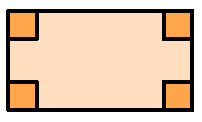### Home > CC1MN > Chapter 9 Unit 9 > Lesson CC1: 9.1.2 > Problem9-28

9-28.

Draw an example of each of the following shapes. If you need help, click each one for the glossary information.

1. rectangle

What are the characteristics of a rectangle?

A rectangle has $4$ sides and $4$ ninety degree angles.1. square

What are the characteristics of a square?

A square has $4$ sides of equal length and $4$ ninety degree angles.

1. parallelogram

What are the characteristics of a parallelogram?

A parallelogram has $4$ sides with two pairs of parallel sides.

1. trapezoid

What are the characteristics of a trapezoid?

A trapezoid is a quadrilateral with at least one pair of parallel sides.

1. scalene triangle

What are the characteristics of a scalene triangle?

A scalene triangle has no congruent sides.

1. right triangle

What are the characteristics of a right triangle?

A right triangle has one right angle.